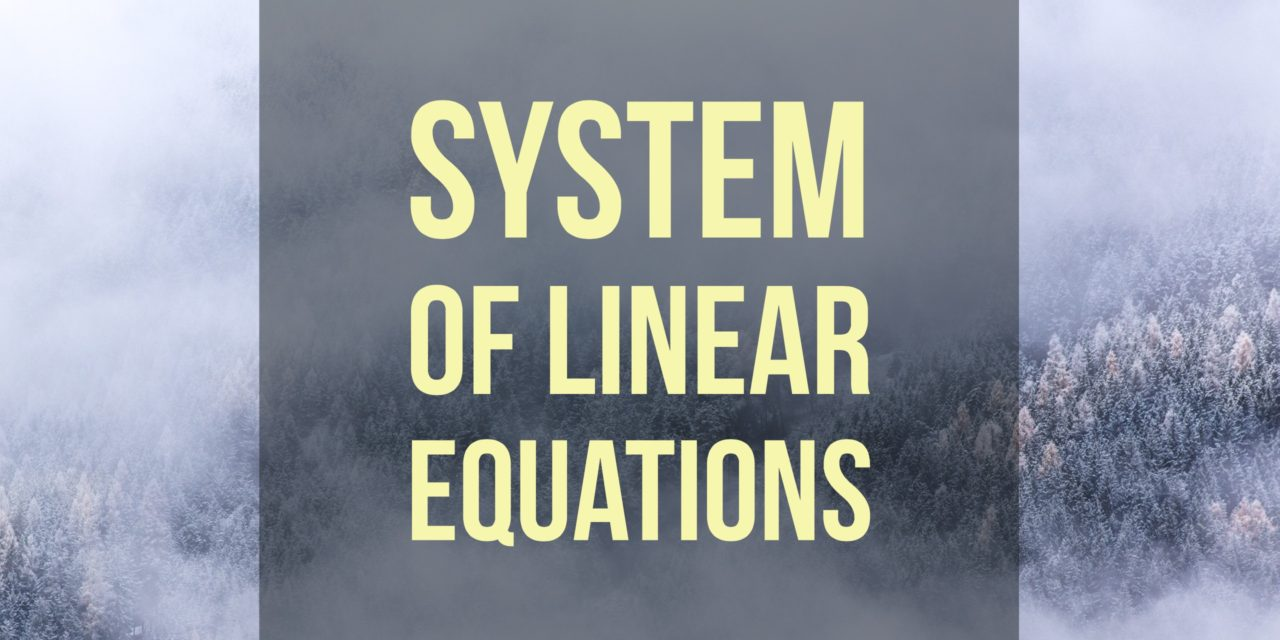# Solve a System of Linear Equations by Gauss-Jordan Elimination## Problem 27

Solve the following system of linear equations using Gauss-Jordan elimination.
\begin{align*}
6x+8y+6z+3w &=-3 \\
6x-8y+6z-3w &=3\\
8y \,\,\,\,\,\,\,\,\,\,\,- 6w &=6
\end{align*}Add to solve later

### We use the following notation.

Elementary row operations.

The three elementary row operations on a matrix are defined as follows.

(1) Interchanging two rows:

$R_i \leftrightarrow R_j$ interchanges rows $i$ and $j$.

(2) Multiplying a row by a non-zero scalar (a number):

$tR_i$ multiplies row $i$ by the non-zero scalar (number) $t$.

(3) Adding a multiple of one row to another row:

$R_j+tR_i$ adds $t$ times row $i$ to row $j$.

## Solution.

The augmented matrix of the system is
$A=\left[ \begin{array}{rrrr|r} 6 & 8 & 6 & 3 & -3 \\ 6 & -8 & 6 & -3 & 3\\ 0 & 8 & 0 & -6 & 6 \end{array} \right].$ We apply elementary row operations as follows to reduce the system to a matrix in reduced row echelon form.

$A \xrightarrow{R_2 – R_1} \left[\begin{array}{rrrr|r} 6 & 8 & 6 & 3 & -3 \\ 0 & -16 & 0 & -6 & 6\\ 0 & 8 & 0 & -6 & 6 \end{array}\right] \xrightarrow[R_2+2R_3]{R_1-R_3} \left[\begin{array}{rrrr|r} 6 & 0 & 6 & 9 & -9 \\ 0 & 0 & 0 & -18 & 18\\ 0 & 8 & 0 & -6 & 6 \end{array}\right]$ $\xrightarrow[\frac{1}{2}R_3]{\frac{1}{3}R_1, \frac{-1}{18}R_2} \left[\begin{array}{rrrr|r} 2 & 0 & 2 & 3 & -3 \\ 0 & 0 & 0 & 1 & -1\\ 0 & 4 & 0 & -3 & 3 \end{array}\right] \xrightarrow[R_3+3R_2]{R_1-3R_2} \left[\begin{array}{rrrr|r} 2 & 0 & 2 & 0 & 0 \\ 0 & 0 & 0 & 1 & -1\\ 0 & 4 & 0 & 0 & 0 \end{array}\right]$

$\xrightarrow[\frac{1}{4}R_3]{\frac{1}{2}R_1} \left[\begin{array}{rrrr|r} 1 & 0 & 1 & 0 & 0 \\ 0 & 0 & 0 & 1 & -1\\ 0 & 1 & 0 & 0 & 0\end{array}\right] \xrightarrow{R_2 \leftrightarrow R_3} \left[\begin{array}{rrrr|r} 1 & 0 & 1 & 0 & 0 \\ 0 & 1 & 0 & 0 & 0 \\ 0 & 0 & 0 & 1 & -1 \end{array}\right]$ The last matrix is in reduced row echelon form.
The corresponding system of linear equations is
\begin{align*}
x+z &=0\\
y&=0 \\
w&=-1
\end{align*}

Let $z=t$ be a free variable. Then the solution is $(x,y,z,w)=(-t,0,t,-1)$ for any number $t$.

### Similar Problem.

Another similar problem is Solving a system of linear equations using Gaussian elimination.
The instruction of the problem says to use Gaussian elimination, but try to solve it using Gauss-Jordan elimination as well.Add to solve later

### 1 Response

1. 01/11/2017

[…] For a similar problem, you may want to check out Solve a system of linear equations by Gauss-Jordan elimination. […]

This site uses Akismet to reduce spam. Learn how your comment data is processed.

###### More in Linear Algebra##### A Matrix is Invertible If and Only If It is Nonsingular

In this problem, we will show that the concept of non-singularity of a matrix is equivalent to the concept of...

Close